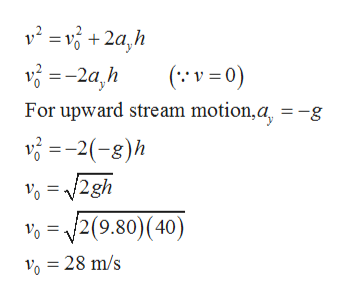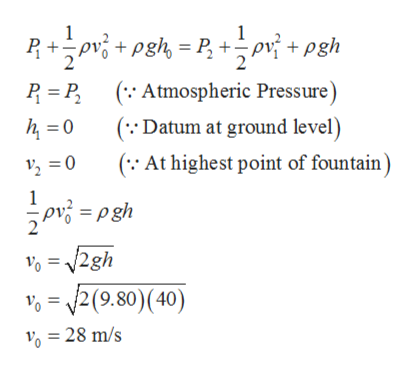Question
7 views

Old Faithful geyser in Yellowstone Park erupts at approximately 1-hour intervals, and the height of the fountain reaches 40.0 m (Fig. P9.47). (a) Consider the rising stream as a series of separate drops. Analyze the free-fall motion of one of the drops to determine the speed at which the water leaves the ground. (b) Treat the rising stream as an ideal fluid in streamline flow. Use Bernoulli’s equation to determine the speed of the water as it leaves ground level. (c) What is the pressure (above atmospheric pressure) in the heated underground chamber 175 m below the vent? You may assume the chamber is large compared with the geyser vent.

check_circle

Step 1

Part (a):

Given,

Step 2

Velocity will be zero at highest height of fountain. By using equation of kinematics we can calculate initial speed of stream as follows,help_outlineImage Transcriptionclosev² = v + 2a,h (:'v = 0) v =-2a,h For upward stream motion,a, = -g v =-2(-8)h V% = /2gh V% = /2(9.80)(40) V, = 28 m/s fullscreen
Step 3

Part (b):

Since pressure acting on stream will be atmospheric pressure only at ground level as well as  highest level and assuming gro...help_outlineImage TranscriptioncloseSor; + pgh, = P, +÷pv + pgh P = P, (:: Atmospheric Pressure) (::Datum at ground level) h = 0 (:· At highest point of fountain) v, = 0 ovi = pgh Vo = V2gh V, = /2(9.80)(40) v, = 28 m/s fullscreen

### Want to see the full answer?

See Solution

#### Want to see this answer and more?

Solutions are written by subject experts who are available 24/7. Questions are typically answered within 1 hour.*

See Solution
*Response times may vary by subject and question.
Tagged in

### Fluid Mechanics